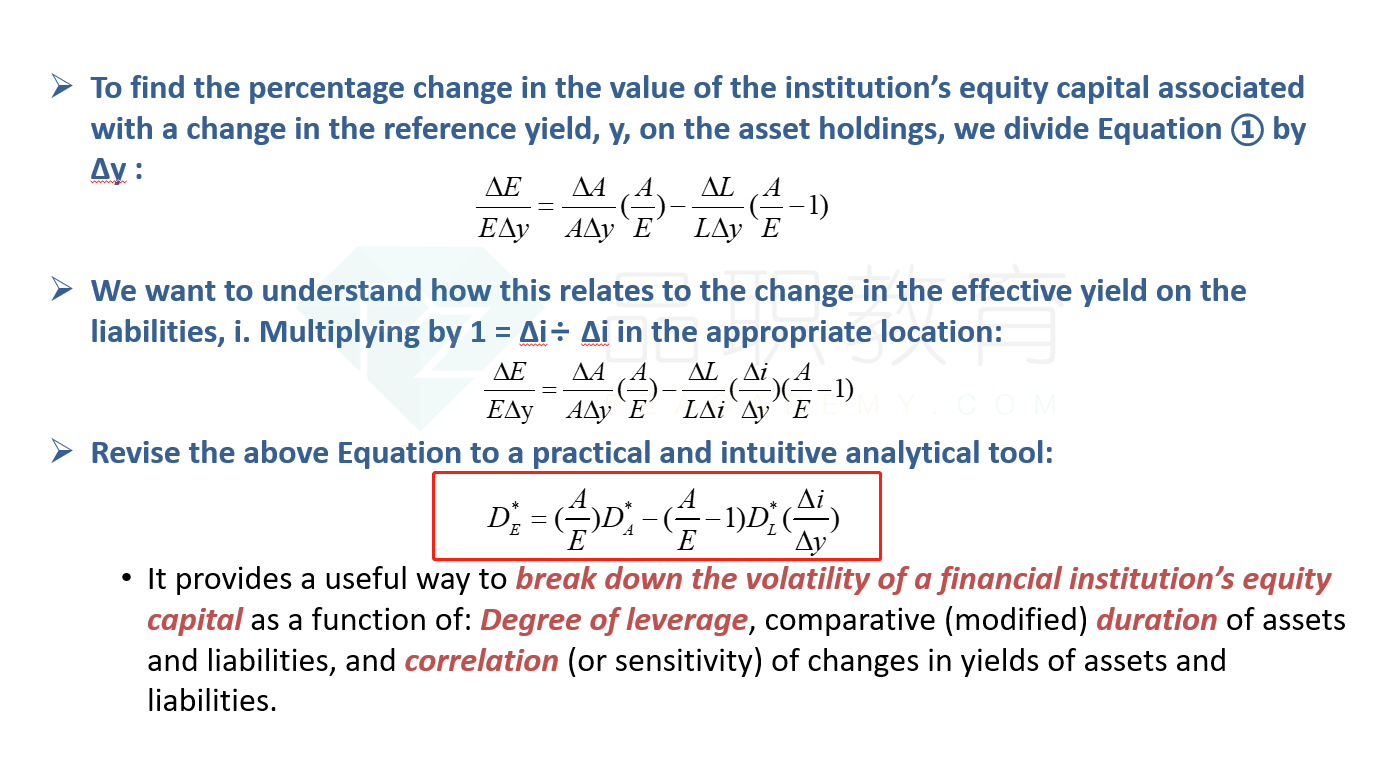Gogo冲 · 2022年11月26日

# equity capital ratio怎么算

NO.PZ2019100901000020

MegaWorld Bancorp has an equity capital ratio for financial assets of 9%. The modified duration of its assets is 2.0 and of its liabilities is 1.5. Over small changes, the yield on liabilities is expected to move by 85 bps for every 100 bps of yield change in its asset portfolio.
Compute the modified duration of the bank’s equity capital.

Using Equation 8, A ÷ E = 1/0.09 = 11.11; (A ÷ E) –1 = 10.11; D*A = 2.0; DL* =
1.5; and Δ
i ÷ Δy = 0.85.
Therefore, the modified duration of shareholders’ capital is:

DE* = (11.11 × 2) – (10.11 × 1.50) × 0.85 = 9.33

###### 1 个答案lynn_品职助教 · 2022年11月28日

equity capital ratio是二级财务里的一个考点，就是E/A，一般是要资本总额除以风险加权资产来计算的。----------------------------------------------